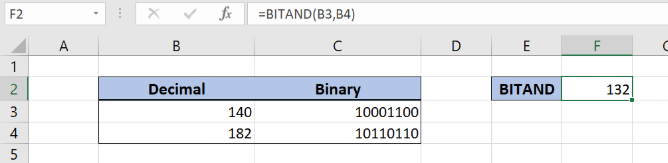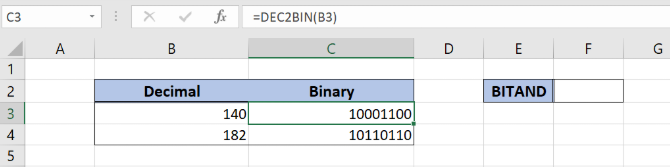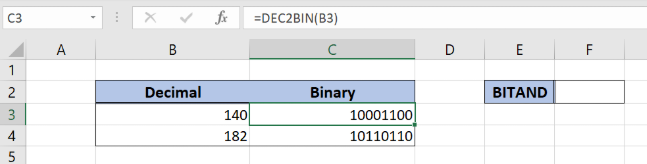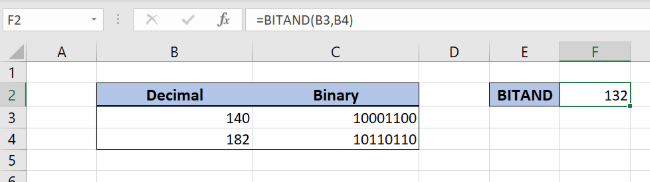Get instant live expert help with Excel or Google Sheets“My Excelchat expert helped me in less than 20 minutes, saving me what would have been 5 hours of work!”

#### Post your problem and you'll get expert help in seconds

Your message must be at least 40 characters
Our professional experts are available now. Your privacy is guaranteed.

# How to Use the BITAND Function in Excel

The BITAND function applies the AND operation on two decimal number. In the AND operation, the bitwise and is applied. This step by step tutorial will assist all levels of Excel users in understanding the binary conversion and the BITAND function.Figure 1. The result of the function

## Conversion of the decimal to binary

Having in mind that the BITAND function operates with the binary numbers, we will briefly explain how to convert decimal to binary.Figure 2. Conversion of decimal numbers to binary

In Figure 2, we can see that the binary value of 140 is 10001100. The function for the conversion is DEC2BIN. The function takes only one parameter, a decimal number, and return a number in a binary format.

## Syntax of the BITAND Formula

The generic formula for the BITAND function is:

`=BITAND(number1, number2)`

The parameters of the BITAND function are:

• Number1 and number2 – decimal numbers that we want to apply bitwise and.

## Setting up Our Data for the BITAND FunctionFigure 3. Data that we will use in the BITAND example

Let’s look at the structure of the data we will use. In the cells B3 and B4 we have the input number. We want to get the result of the BITAND function in the cell F2. The binary values in C3 and C4 are here to help us to understand the function.

## Performing bitwise AND on two numbers

In our example, we want to apply bitwise AND on 140 and 182. The binary formats of these numbers are 10001100 and 10110110.Figure 4. Using the BITAND function

The formula looks like:

`=BITAND(B3, B4)`

The parameter number1 is the cell B3, while the parameter number2 is the cell B4. The function applies AND on numbers’ digits (1 and 1 is 1, 0 and 0 is 0, 0 and 1 is 0, etc.). The result of this operation is 10000100, which is 132 in decimal format. This is the result of the function in the cell F2.

To apply the BITAND function, we need to follow these steps:

• Select cell F2 and click on it
• Insert the formula: `=BITAND(B3, B4)`
• Press enter

Most of the time, the problem you will need to solve will be more complex than a simple application of a formula or function. If you want to save hours of research and frustration, try our live Excelchat service! Our Excel Experts are available 24/7 to answer any Excel question you may have. We guarantee a connection within 30 seconds and a customized solution within 20 minutes.

### Did this post not answer your question? Get a solution from connecting with the expert.Another blog reader asked this question today on Excelchat:## Subscribe to Excelchat.coAnother blog reader asked this question today on Excelchat: